## 一，精确率、召回率与F1

### 1.2，精确率、召回率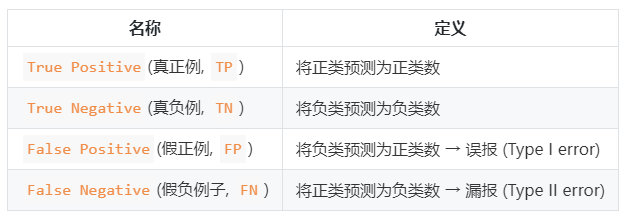• 查准率（精确率）P=TP/(TP+FP)P=TP/(TP+FP)
• 查全率（召回率）R=TP/(TP+FN)R=TP/(TP+FN)

### 1.3，F1 分数F1 度量的一般形式：​，能让我们表达出对查准率/查全率的偏见，​ 计算公式如下：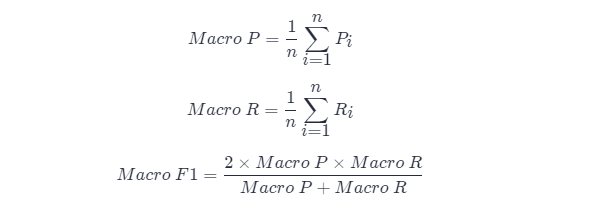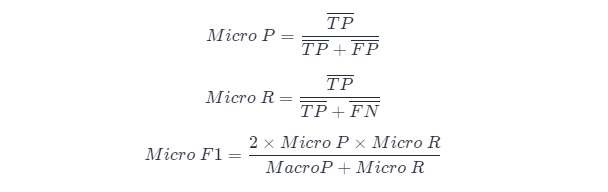### 1.4，PR 曲线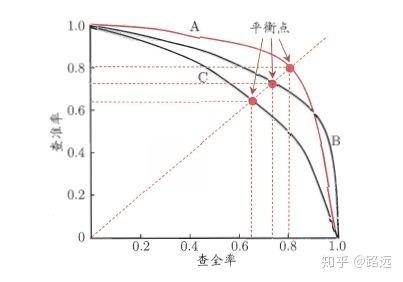### 1.5，ROC 曲线与 AUC 面积

• PR 曲线是以 Recall 为横轴，Precision 为纵轴；而 ROC 曲线则是以 FPR 为横轴，TPR 为纵轴**。P-R 曲线越靠近右上角性能越好。PR 曲线的两个指标都聚焦于正例
• PR 曲线展示的是 Precision vs Recall 的曲线，ROC 曲线展示的是 FPR（x 轴：False positive rate） vs TPR（True positive rate, TPR）曲线。
1. [ ] ROC 曲线
2. [ ] AUC 面积

## 二，AP 与 mAP

### 2.1，AP 与 mAP 指标理解

AP 衡量的是训练好的模型在每个类别上的好坏，mAP 衡量的是模型在所有类别上的好坏，得到 AP 后 mAP 的计算就变得很简单了，就是取所有 AP 的平均值。AP 的计算公式比较复杂（所以单独作一章节内容），详细内容参考下文。

mAP 这个术语有不同的定义。此度量指标通常用于信息检索、图像分类和目标检测领域。然而这两个领域计算 mAP 的方式却不相同。这里我们只谈论目标检测中的 mAP 计算方法。

mAP 常作为目标检测算法的评价指标，具体来说就是，对于每张图片检测模型会输出多个预测框（远超真实框的个数），我们使用 IoU (Intersection Over Union，交并比)来标记预测框是否预测准确。标记完成后，随着预测框的增多，查全率 R 总会上升，在不同查全率 R 水平下对准确率 P 做平均，即得到 AP，最后再对所有类别按其所占比例做平均，即得到 mAP 指标。

### 2.2，近似计算AP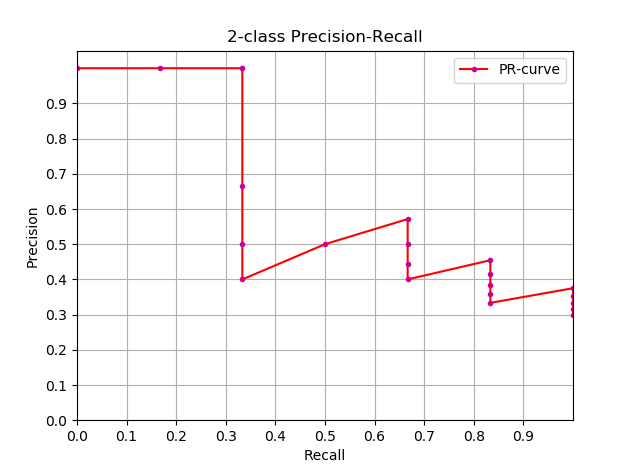• 近似计算 AP (approximated average precision)，这种计算方式是 approximated 形式的；
• 很显然位于一条竖直线上的点对计算 AP 没有贡献；
• 这里 N 为数据总量，k 为每个样本点的索引， Δr(k)=r(k)−r(k−1)。

``````import numpy as np
import matplotlib.pyplot as plt
class_names = ["car", "pedestrians", "bicycle"]
def draw_PR_curve(predict_scores, eval_labels, name, cls_idx=1):
"""calculate AP and draw PR curve, there are 3 types
Parameters:
@all_scores: single test dataset predict scores array, (-1, 3)
@all_labels: single test dataset predict label array, (-1, 3)
@cls_idx: the serial number of the AP to be calculated, example: 0,1,2,3...
"""
# print('sklearn Macro-F1-Score:', f1_score(predict_scores, eval_labels, average='macro'))
global class_names
fig, ax = plt.subplots(nrows=1, ncols=1, figsize=(15, 10))
# Rank the predicted scores from large to small, extract their corresponding index(index number), and generate an array
idx = predict_scores[:, cls_idx].argsort()[::-1]
eval_labels_descend = eval_labels[idx]
pos_gt_num = np.sum(eval_labels == cls_idx) # number of all gt
predict_results = np.ones_like(eval_labels)
tp_arr = np.logical_and(predict_results == cls_idx, eval_labels_descend == cls_idx) # ndarray
fp_arr = np.logical_and(predict_results == cls_idx, eval_labels_descend != cls_idx)
tp_cum = np.cumsum(tp_arr).astype(float) # ndarray, Cumulative sum of array elements.
fp_cum = np.cumsum(fp_arr).astype(float)
precision_arr = tp_cum / (tp_cum + fp_cum) # ndarray
recall_arr = tp_cum / pos_gt_num
ap = 0.0
prev_recall = 0
for p, r in zip(precision_arr, recall_arr):
ap += p * (r - prev_recall)
# pdb.set_trace()
prev_recall = r
print("------%s, ap: %f-----" % (name, ap))
fig_label = '[%s, %s] ap=%f' % (name, class_names[cls_idx], ap)
ax.plot(recall_arr, precision_arr, label=fig_label)
ax.legend(loc="lower left")
ax.set_title("PR curve about class: %s" % (class_names[cls_idx]))
ax.set(xticks=np.arange(0., 1, 0.05), yticks=np.arange(0., 1, 0.05))
ax.set(xlabel="recall", ylabel="precision", xlim=[0, 1], ylim=[0, 1])
fig.savefig("./pr-curve-%s.png" % class_names[cls_idx])
plt.close(fig)``````

### 2.3，插值计算 AP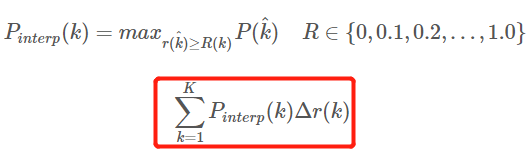• 这是通常意义上的 11 points_Interpolated 形式的 AP，选取固定的 0,0.1,0.2,…,1.00,0.1,0.2,…,1.0 11 个阈值，这个在 PASCAL2007 中使用
• 这里因为参与计算的只有 11 个点，所以 K=11，称为 11 points_Interpolated，k 为阈值索引
• Pinterp​(k) 取第 k 个阈值所对应的样本点之后的样本中的最大值，只不过这里的阈值被限定在了 0,0.1,0.2,…,1.00,0.1,0.2,…,1.0 范围内。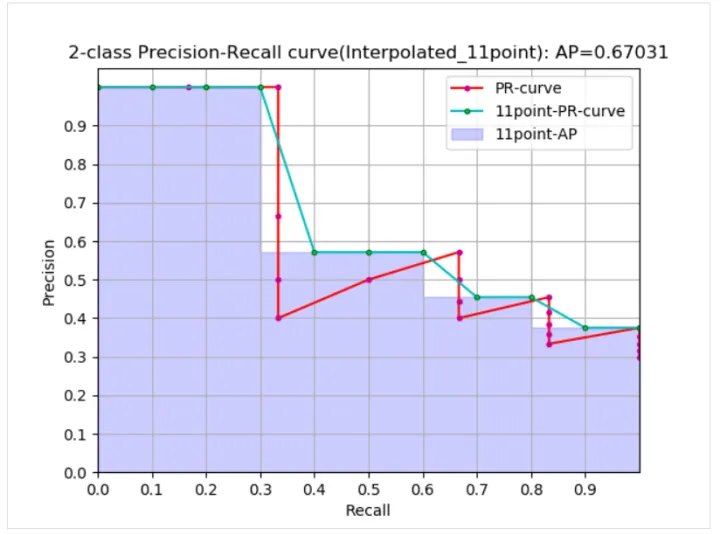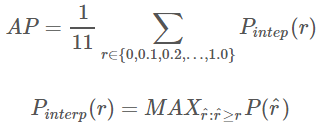1、在给定 recal 和 precision 的条件下计算 AP：

``````def voc_ap(rec, prec, use_07_metric=False):
"""
ap = voc_ap(rec, prec, [use_07_metric])
Compute VOC AP given precision and recall.
If use_07_metric is true, uses the
VOC 07 11 point method (default:False).
"""
if use_07_metric:
# 11 point metric
ap = 0.
for t in np.arange(0., 1.1, 0.1):
if np.sum(rec >= t) == 0:
p = 0
else:
p = np.max(prec[rec >= t])
ap = ap + p / 11.
else:
# correct AP calculation
# first append sentinel values at the end
mrec = np.concatenate(([0.], rec, [1.]))
mpre = np.concatenate(([0.], prec, [0.]))
# compute the precision envelope
for i in range(mpre.size - 1, 0, -1):
mpre[i - 1] = np.maximum(mpre[i - 1], mpre[i])
# to calculate area under PR curve, look for points
# where X axis (recall) changes value
i = np.where(mrec[1:] != mrec[:-1])
# and sum (\Delta recall) * prec
ap = np.sum((mrec[i + 1] - mrec[i]) * mpre[i + 1])
return ap``````

2、给定目标检测结果文件和测试集标签文件 xml 等计算 AP：

``````def parse_rec(filename):
""" Parse a PASCAL VOC xml file
Return : list, element is dict.
"""
tree = ET.parse(filename)
objects = []
for obj in tree.findall('object'):
obj_struct = {}
obj_struct['name'] = obj.find('name').text
obj_struct['pose'] = obj.find('pose').text
obj_struct['truncated'] = int(obj.find('truncated').text)
obj_struct['difficult'] = int(obj.find('difficult').text)
bbox = obj.find('bndbox')
obj_struct['bbox'] = [int(bbox.find('xmin').text),
int(bbox.find('ymin').text),
int(bbox.find('xmax').text),
int(bbox.find('ymax').text)]
objects.append(obj_struct)
return objects
def voc_eval(detpath,
annopath,
imagesetfile,
classname,
cachedir,
ovthresh=0.5,
use_07_metric=False):
"""rec, prec, ap = voc_eval(detpath,
annopath,
imagesetfile,
classname,
[ovthresh],
[use_07_metric])
Top level function that does the PASCAL VOC evaluation.
detpath: Path to detections result file
detpath.format(classname) should produce the detection results file.
annopath: Path to annotations file
annopath.format(imagename) should be the xml annotations file.
imagesetfile: Text file containing the list of images, one image per line.
classname: Category name (duh)
cachedir: Directory for caching the annotations
[ovthresh]: Overlap threshold (default = 0.5)
[use_07_metric]: Whether to use VOC07's 11 point AP computation
(default False)
"""
# assumes detections are in detpath.format(classname)
# assumes annotations are in annopath.format(imagename)
# assumes imagesetfile is a text file with each line an image name
# cachedir caches the annotations in a pickle file
if not os.path.isdir(cachedir):
os.mkdir(cachedir)
cachefile = os.path.join(cachedir, '%s_annots.pkl' % imagesetfile)
with open(imagesetfile, 'r') as f:
imagenames = [x.strip() for x in lines]
if not os.path.isfile(cachefile):
recs = {}
for i, imagename in enumerate(imagenames):
recs[imagename] = parse_rec(annopath.format(imagename))
if i % 100 == 0:
i + 1, len(imagenames)))
# save
print('Saving cached annotations to {:s}'.format(cachefile))
with open(cachefile, 'wb') as f:
pickle.dump(recs, f)
else:
with open(cachefile, 'rb') as f:
try:
except:
# extract gt objects for this class
class_recs = {}
npos = 0
for imagename in imagenames:
R = [obj for obj in recs[imagename] if obj['name'] == classname]
bbox = np.array([x['bbox'] for x in R])
difficult = np.array([x['difficult'] for x in R]).astype(np.bool)
det = [False] * len(R)
npos = npos + sum(~difficult)
class_recs[imagename] = {'bbox': bbox,
'difficult': difficult,
'det': det}
detfile = detpath.format(classname)
with open(detfile, 'r') as f:
splitlines = [x.strip().split(' ') for x in lines]
image_ids = [x for x in splitlines]
confidence = np.array([float(x) for x in splitlines])
BB = np.array([[float(z) for z in x[2:]] for x in splitlines])
nd = len(image_ids)
tp = np.zeros(nd)
fp = np.zeros(nd)
if BB.shape > 0:
# sort by confidence
sorted_ind = np.argsort(-confidence)
sorted_scores = np.sort(-confidence)
BB = BB[sorted_ind, :]
image_ids = [image_ids[x] for x in sorted_ind]
# go down dets and mark TPs and FPs
for d in range(nd):
R = class_recs[image_ids[d]]
bb = BB[d, :].astype(float)
ovmax = -np.inf
BBGT = R['bbox'].astype(float)
if BBGT.size > 0:
# compute overlaps
# intersection
ixmin = np.maximum(BBGT[:, 0], bb)
iymin = np.maximum(BBGT[:, 1], bb)
ixmax = np.minimum(BBGT[:, 2], bb)
iymax = np.minimum(BBGT[:, 3], bb)
iw = np.maximum(ixmax - ixmin + 1., 0.)
ih = np.maximum(iymax - iymin + 1., 0.)
inters = iw * ih
# union
uni = ((bb - bb + 1.) * (bb - bb + 1.) +
(BBGT[:, 2] - BBGT[:, 0] + 1.) *
(BBGT[:, 3] - BBGT[:, 1] + 1.) - inters)
overlaps = inters / uni
ovmax = np.max(overlaps)
jmax = np.argmax(overlaps)
if ovmax > ovthresh:
if not R['difficult'][jmax]:
if not R['det'][jmax]:
tp[d] = 1.
R['det'][jmax] = 1
else:
fp[d] = 1.
else:
fp[d] = 1.
# compute precision recall
fp = np.cumsum(fp)
tp = np.cumsum(tp)
rec = tp / float(npos)
# avoid divide by zero in case the first detection matches a difficult
# ground truth
prec = tp / np.maximum(tp + fp, np.finfo(np.float64).eps)
ap = voc_ap(rec, prec, use_07_metric)
return rec, prec, ap``````

### 2.4，mAP 计算方法

（1）在 VOC2007，只需要选取当 Recall>=0,0.1,0.2,...,1Recall>=0,0.1,0.2,...,1 共 11 个点时的 Precision 最大值，然后 APAP 就是这 11 个 Precision 的平均值，mAPmAP 就是所有类别 APAP 值的平均。VOC 数据集中计算 APAP 的代码（用的是插值计算方法，代码出自py-faster-rcnn仓库

（2）在 VOC2010 及以后，需要针对每一个不同的 Recall 值（包括 0 和 1），选取其大于等于这些 Recall 值时的 Precision 最大值，然后计算 PR 曲线下面积作为 AP 值，mAPmAP 就是所有类别 AP 值的平均。

（3）COCO 数据集，设定多个 IOU 阈值（0.5-0.95, 0.05 为步长），在每一个 IOU 阈值下都有某一类别的 AP 值，然后求不同 IOU 阈值下的 AP 平均，就是所求的最终的某类别的 AP 值。

## 三，目标检测度量标准汇总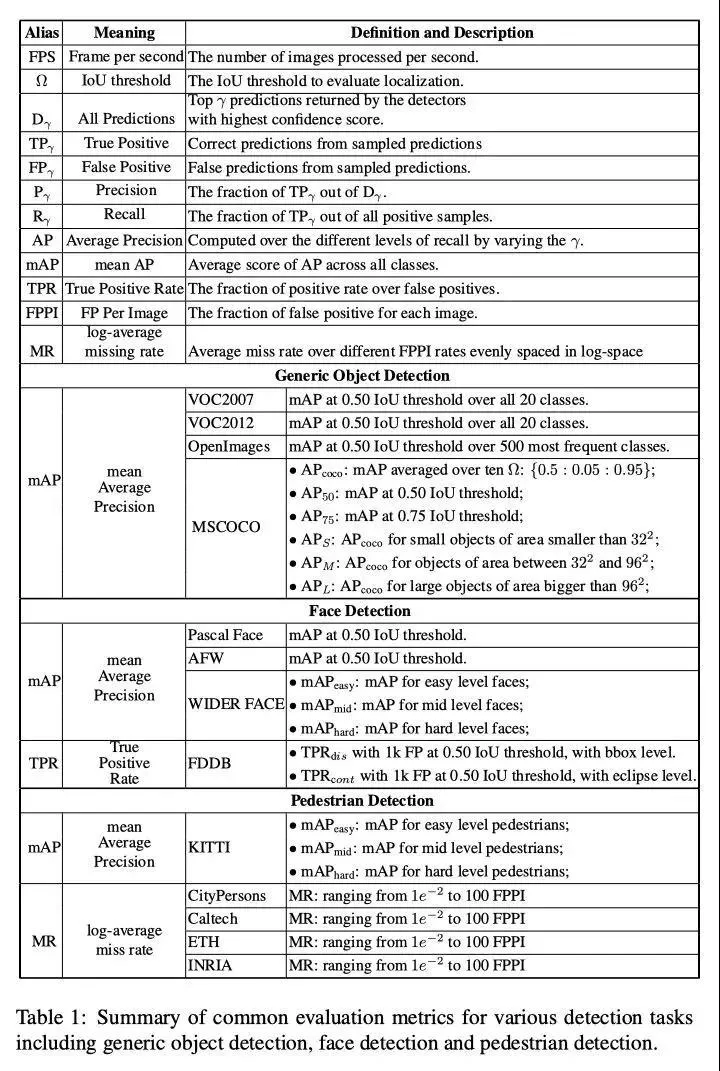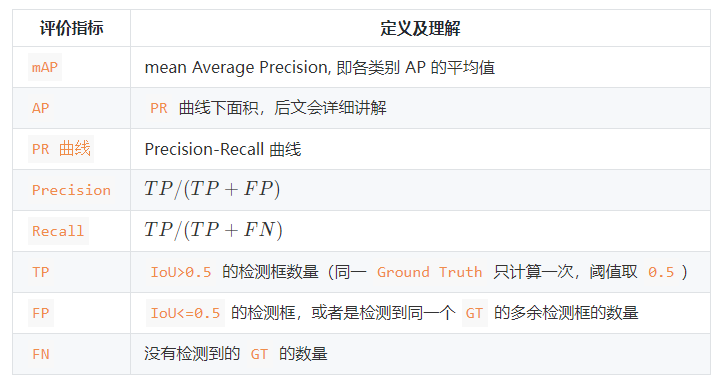## 四，参考资料